##CS 660: Combinatorial Algorithms BB[ alpha ] trees

[To Lecture Notes Index]
San Diego State University -- This page last updated October 24, 1995## Reference

Mehlhorn, Data Structures and Algorithms 1: Sorting and Searching, pages 189-199

## Weight-Balanced Trees

Let Alpha be a real such that 1/4 < Alpha <= 1 -Let T be a binary search tree

Let Tl (Tr ) be the left (right) subtree of the root of T

Definitions

The root balance of T, Rho , is given by:
Rho(T) = | Tl | / |T | = 1 - | Tr | / | T |

where | T | = number of leaves of tree T

A tree T is of bounded balance Alpha ,
if for every subtree T' of T we have:
Alpha <= Rho(T' ) <= 1 - Alpha

BB[ Alpha ] is the set of all trees of bounded balance Alpha
Example of BB[ Alpha ] tree
Alpha <= 1/3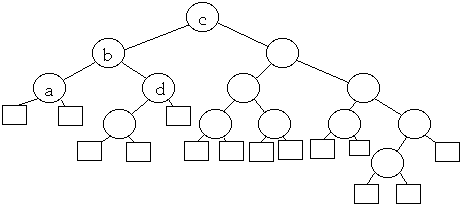Node Root balance a 1/2 b 2/5 c 5/14 d 2/3
Let bi denote the depth of node xi in tree T. The average path length of T is given by: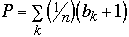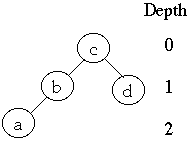Theorem 2 Let T be a BB[ Alpha ] with n nodes. Then

a)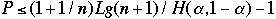b)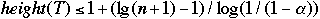ifthen theorem 2 states
P <= 1.15(1 + 1/n) lg( n+1) - 1
and
height(T) <= 2 lg( n +1 ) - 1

### Rotations and Root BalanceLet a, b ,c denote the number of leaves in the subtrees shown above.

Let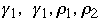denote the root balance of the subtrees indicated above. We have by definition:So: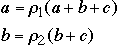This gives: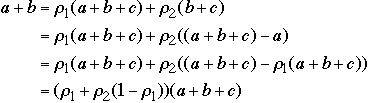Using the above we get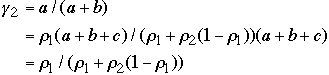and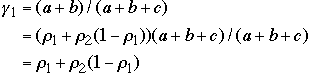Thus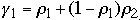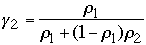Rotations and Root Balance
Double Rotation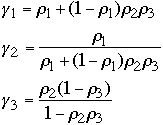### Performance

Lemma
Let.
Let T' be a BB[ Alpha ] tree.
Let T be result of adding (deleting) a node from T'.
Assume that Tl and Tr are BB[ Alpha ] trees.
Assume that T is no longer a BB[ Alpha ] tree.
Then a single or double rotation will balance T

Theorem.
Let. Then there is a constant c such that the total number of single and double rotations required in a sequence of M insertions and deletions into an empty BB[ Alpha ] tree is <= cM
For = 3/11 the prove gives the value of c = 19.59

Experiments suggest that c is near 1

Theorem.
Let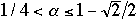. Then insert, access, delete takes O(N) in a BB[ Alpha ] tree with N leaves